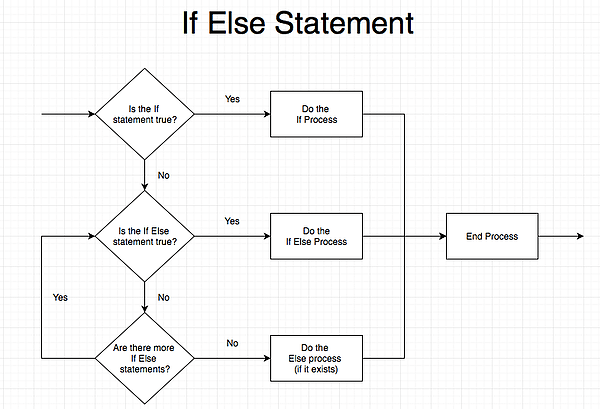VBA If Else

Macro processes only run if the criteria is true

VBA If Else statements

Building a VBA If Else statement is quite similar to building a nested if formula inside Excel. The benefit of using VBA instead is that it’s much easier to follow, since nested Ifs tend to complicate with multiple parenthesis enclosures. In a VBA if statement, each If clause is separate from the other, and is instead laid out in order of priority from top to bottom.

If statement structure in VBA

If Range(“A5”) = “YES” Then

Range(“A5”).Value = “NO”

End If

• If statements begin with an If [CRITERIA] Then statement
• If the user does not wish to add additional statements, the if statement is closed by an End If
• In the above example, no false statement is present. Thus, if Cell A5 is not YES, VBA will simply skip this statement and move on to whatever code follows it. If Cell A5 is YES, the process contained in the If statement will be run.

If Range(“A5”) <= 100 Then

Range(“A6”).Value = “Decent Performance”

Else If Range(“A5”) > 100 Then

Range(“A6”).Value = “Great Performance”

End If

• If the user does want to add additional statements assuming the first If … Then is false, then the first if … then the process should be immediately followed by the Else If … Then statement.
• There can be multiple Else If … Then clauses in a VBA if statement, as long as each Else If … criteria is mutually exclusive from other Else If or If criteria.
• End If should close the last Else If … Then statement.
• In the above example, if Cell A5 is 91, then Cell A6 will be set to Decent Performance.

If Range(“A5”) <= 50 Then

Else If Range (“A5”) <= 80 Then

Else

End If

• The user can also elect to include one Else statement, which will activate if all other If and Else If statements are false.
• Else statements are not followed by Then, unlike If … Then and Else If … Then statements.
• End If should close an Else statement, and only the Else statement, if it is used.
• In the above example, if Cell A5 is 91, then Cell A6 will be set to Outstanding Grade.
• Else statements act as a “catch-all” and will ensure that one process is run, even if all the other If statements are false.
• Excluding an else statement may result in the entire if to end if statement being skipped over, if all criteria are false.

An If formula in Excel worksheets will need a clause to perform if the statement is true and one to perform if the statement is false. In contrast, a VBA if statement only needs code to perform if the statement is true. The section defining what to perform if that statement is false is optional. If the VBA statement turns out to be false and no process is defined for a false statement, then VBA will simply skip the if statement and move on to the next process.This is taught in CFI’s VBA modeling course.

Uses Of The VBA If Else Statement

The VBA If Else statement allows you to set up “gates” that only activate or open when a certain criterion is met. If the criteria are not met, a VBA macro will simply skip over that portion of code. In financial modelling, this may be useful for creating a macro that only runs when a net income cell is positive, for example, or if a stock price exceeds a certain limit.

Because of the structure of the If statement and the ability to set different code depending on the value in the criteria, the If statement is also great for creating reactive macros that perform differently based on the situation. For example, a macro can be written for different cases of a valuation. An if statement can be used to perform a different action if the valuation follows the base case, the downside, or the upside.

If statements can also be used in conjunction with loops. In other words, If statements can contain loops that repeat a certain process. On the other hand, loops can also contain If statements, and will only perform that part of the loop if the stated criteria are met.

• VBA Referencing
• VBA Data Types
• VBA Do Loops
• VBA Methods
• VBA Modeling Course Online

Free Excel Tutorial

To master the art of Excel, check out CFI’s FREE Excel Crash Course, which teaches you how to become an Excel power user.  Learn the most important formulas, functions, and shortcuts to become confident in your financial analysis.

Launch CFI’s Free Excel Course now to take your career to the next level and move up the ladder!